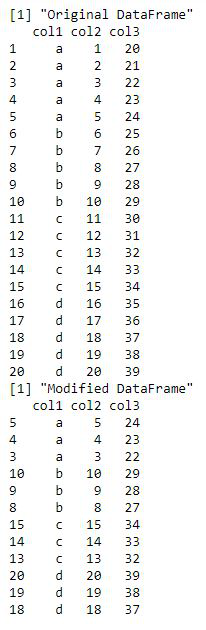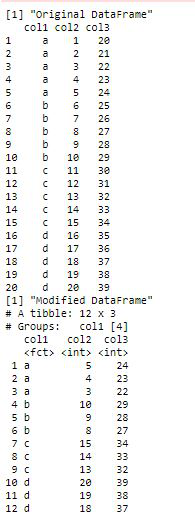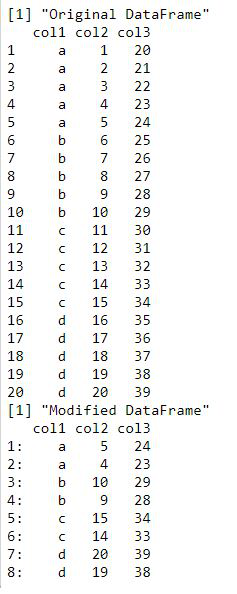Select Top N Highest Values by Group in R

• Last Updated : 26 Oct, 2021

In this article, we are going to see how to select the Top Nth highest value by the group in R language.

Method 1: Using Reduce method

The dataframe can be ordered by group in descending order of their values by the order method. The corresponding dataframe is then accessed using the indexing method by taking the order function’s output in the form of row indexes.

Syntax: order(vec, decreasing = TRUE)

Arguments :

• vec – The dataframe column name to arrange in descending order
• decreasing – The flag to set data in descending order

The Reduce method in base R can also be used to select top n highest rows from each group in a dataframe. This method takes as input a function f of two arguments and also a list or vector vec, which is to be reduced using the function f. The function f is rbind method, which is used to bind the rows together to form a dataframe. The by() method in R is used to apply a function to specified subsets of a dataframe. The first argument of this method takes up the data and second parameter is by which the function is applied and third parameter is the function. Here, the head is used as the function specified using the third argument of the method call. It is used to specify the the n rows group wise from the dataframe.

Syntax: by(df, df\$col-name, FUN)

Arguments :

• df – The dataframe to apply the functions on
• FUN – The function to be applied

The combined function application can be summarized as follows :

Reduce(rbind,by())

Code:

R

 # creating dataframedata_frame <- data.frame(col1 = rep(letters[1:4], each = 5),                         col2 = 1:20,                         col3 = 20:39)print("Original DataFrame")print(data_frame)  # sorting the data by the column# required in descending orderdata_sorted <- data_frame[order(data_frame\$col2,                                decreasing = TRUE), ]  # select top 3 values from each groupdata_mod <- Reduce(rbind,                                                    by(data_sorted,                       data_sorted["col1"],                       head,                       n = 3))  print ("Modified DataFrame")print (data_mod)

Output:Method 2: Using dplyr package

The dplyr package in R is used to perform mutations and data manipulations in R. It is particularly useful for working with dataframes and data tables. The package can be downloaded and installed into the working directory using the following command :

install.packages(“dplyr”)

A sequence of methods are available in this package which are used to select top n rows from each group in a dataframe. Initially, the arrange() method is invoked to arrange the data of the dataframe in the ascending order or descending order. The descending order is invoked using the desc() method. The column name specified as the argument in this method is used for arranging the data.

arrange(desc(col-name))

This is followed by the application of the group_by method which takes as arguments the set of column names that are used for grouping the data. It may comprise of one or more columns.

group_by(col-name1, col-name2..)

Then slice() method is used to retrieve the top n rows from the dataframe.

slice(1:n)

The output is returned in the form of a tibble containing entire information about the rows returned. The row numbers of the original dataframe are not retained.

Code:

R

 library("dplyr")  # creating dataframedata_frame <- data.frame(col1 = rep(letters[1:4], each = 5),                         col2 = 1:20,                         col3 = 20:39)print("Original DataFrame")print(data_frame)  # sorting the data by the column# required in descending orderdata_mod<- data_frame %>%                                        arrange(desc(col2)) %>%   group_by(col1) %>%  slice(1:3)print("Modified DataFrame")print(data_mod)

Output:Method 3: Using data.table package

The data.table method in R is used to perform data storage and manipulations in a well organized manner. The package can be downloaded and installed into the working directory using the following command :

install.packages(data.table)

The data table can be re-ordered by group in descending order of their values by the order method. The corresponding dataframe is then accessed using the indexing method by taking the order function’s output in the form of row indexes.

Syntax: order(vec, decreasing = TRUE)

Arguments :

Vec – The dataframe column name to arrange in descending order

Decreasing – The flag to set data in descending order

The dataframe can then be converted into a data table using the data.table() method along with the column name to be used in setKey() method. The key attribute contains the column name to group the data by in the data.table.

data.table(df, key = )

Now, the head along with .SD attribute can be used to access the top n rows of each of the taken groups. The by argument contains the grouping column. The head method takes as arguments .SD and integer value n.

df[ , head(.SD, 3), by =]

Code:

R

 library("data.table")  # creating dataframedata_frame <- data.frame(col1 = rep(letters[1:4], each = 5),                         col2 = 1:20,                         col3 = 20:39)print("Original DataFrame")print(data_frame)  # sorting the data in descending order  # Top N highest values by groupdata_mod <- data_frame[order(data_frame\$col2, decreasing = TRUE), ]    # organising the data by groupdata_mod <- data.table(data_mod, key = "col1")  # getting top2 valuesdata_mod <- data_mod[ , head(.SD, 2), by = col1]  # printing modified dataframe                                       print("Modified DataFrame")print(data_mod)

Output:My Personal Notes arrow_drop_up